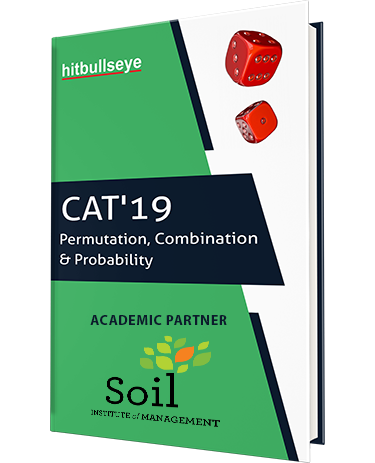# Permutation and Combination and Probability Question Bank

P&C and Probability form important segments of Quantitative section of CAT. You need to practice all the basic P&C tricks, techniques & formulae to  crack CAT level questions. Then, the next step is to implement these techniques & invest a good amount of time in practicing these questions.Download Now